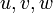# Algebra group structures for dihedral group:D8

(diff) ← Older revision | Latest revision (diff) | Newer revision → (diff)
View algebra group structures for particular groups | View other specific information about dihedral group:D8

The group dihedral group:D8 has at least one (and probably only one?) algebra group structure over field:F2. It does not have any algebra group structure over any other fields.

## Algebra

### Multiplication table (structure constants)

The algebra is a three-dimensional algebra. We can describe it by means of the following multiplication table in terms of structure constants$u,v,w$. The multiplication table is as follows:$u$$v$$w$$u$ 0$w$ 0$v$ 0 0 0$w$ 0 0 0

### Verification of properties

• The algebra is associative: All products of length three or more are zero.
• The algebra is nilpotent: All products of length three or more are zero.
• The algebra group is isomorphic to dihedral group:D8:$1 + w$ is the central element of order 2,$1 + u + v$ is the order four generator of the cyclic maximal subgroup.$1 + u$ and$1 + v$ are reflections outside this subgroup.

### Description as subalgebra of niltriangular matrix Lie algebra

The algebra is the whole of niltriangular matrix Lie algebra:NT(3,2), so dihedral group:D8 is isomorphic to$UT(3,2)$. The explicit description is:$u = \begin{pmatrix} 0 & 1 & 0 \\ 0 & 0 & 0 \\ 0 & 0 & 0 \\\end{pmatrix}, \qquad v = \begin{pmatrix} 0 & 0 & 0 \\ 0 & 0 & 1 \\ 0 & 0 & 0 \\\end{pmatrix}, \qquad w = \begin{pmatrix} 0 & 0 & 1 \\ 0 & 0 & 0 \\ 0 & 0 & 0 \\\end{pmatrix}$